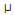# Electronics - Capacitors

### Exercise :: Capacitors - General Questions

56.

Which of the following is the reference vector for parallel RC circuits?

 A. R B. I C. C D. V

Explanation:

No answer description available for this question. Let us discuss.

57.

What do variable capacitors use for dielectric?

 A. ceramic, electrolytic, mica, or paper B. air, ceramic, mica, or plastic C. ceramic, paper, plastic, or mica D. mica, ceramic, plastic, or electrolytic

Explanation:

No answer description available for this question. Let us discuss.

58.

If a charged capacitor is disconnected from a circuit, it will:

 A. immediately discharge B. recharge C. remain charged D. leak the charge

Explanation:

No answer description available for this question. Let us discuss.

59.

What is the total current for a 5 MHz, 1 Vac circuit that consists of a 27 pF capacitor and a 1 kresistor connected in parallel?

 A. 459A B. 647A C. 1.31 mA D. 1.85 mA

Explanation:

No answer description available for this question. Let us discuss.

60.

What is the value of a capacitor that can store two coulombs of charge when 500 volts is applied across its plates?

 A. 4F B. 250F C. 4,000F D. 250 F